#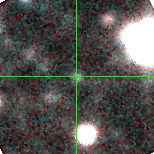M33C-22178 (J013355.78+304831.3)

## Target Notes### Classification: YSG

No blend concerns but marked "X" when signal to noise is too low to get a good PSF fit.

## Plots of Brightness and Color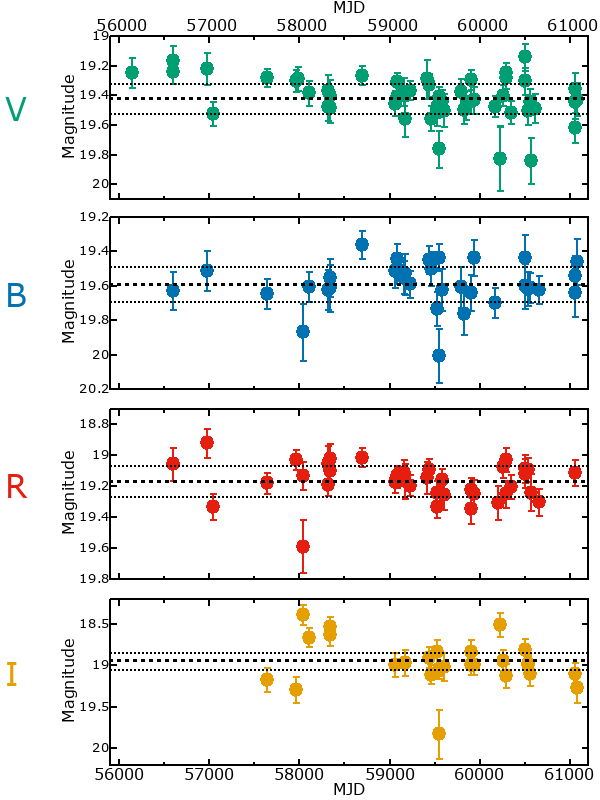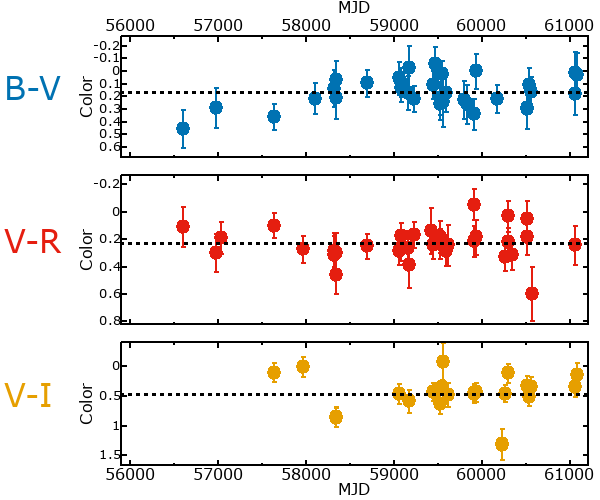## Variability

From the data available in this survey, it is not possible to tell with certainty if this star is variable.

### Correlation factors between bands

If the brightness variation is true variability then there should be a correlation between brightness changes recorded in different filter bands. The following table are the R-squared linear correlation factors calculated for the pairs of bands. The number in parentheses next to the R-squared value is the number of elements used to calculate the correlation factor. The number in square brackets next to the band is the range of magnitudes measured.

V [0.52 mag]B [0.41 mag]R [0.47 mag]
B [0.52 mag]0.22 (14)
R [0.47 mag]0.34 (16)0.64 (14)
I [0.90 mag]0.28 (6)0.53 (5)0.08 (7)

### Magnitude-Magnitude Plots

The plots below are provided to check the values in the table above and also to show any non-linear correlations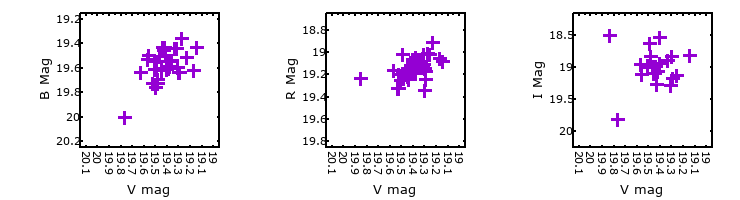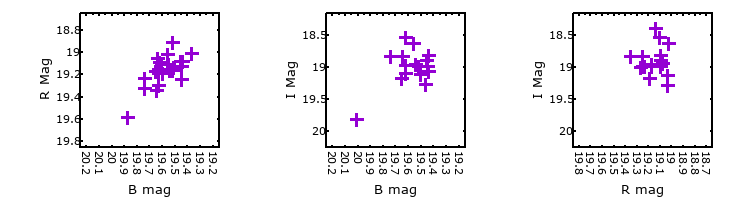### I & R Statistics

Welch & Stetson (1993, AJ, 105, 1813) present a method for varible star detection in CCD images from automated surveys. Data from two bands are compared and two statistics are calculated: R and I.

The I-statistic has an expectation value of zero for stars that are NOT variable. A larger non-zero I-value indicates a higher likelihood that the star is variable. The R statistic indicates how the color of the star changes as the variable grows brighter with R = 1.0 being no change in color.

R and I values have only been calculated if there are more than four (4) matched measurements of brightness in each band.
V
(I|R)
B
(I|R)
R
(I|R)
B
(I|R)
0.43 | 0.59
R
(I|R)
0.92 | 0.911.07 | 0.13
I
(I|R)
-1.47 | 0.720.36 | 0.130.51 | 0.32